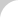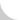The Complete Guide to Chain# 4.1.4 Power Transmission Chain Selection for Slow Speeds

This selection procedure is based on the maximum allowable tension, which is used when the chain speed is less than 50 m/min., and the starting frequency is less than 5 times/day. The selection is done following the flow chart in Figure 4.11.

EXAMPLE: Recalculate the previous example from Basics Section 4.1.3 based upon the selection for slow speed.

• Step 1. Tentatively select RS120 chain, which is one size smaller than RS140, and a 15-tooth sprocket. Then calculate the chain speed.
V = PNn / 1,000 = (38.1 × 15 × 50)/ 1,000 = 28.6 m/min. < 50
According to this speed and starting frequency, case selection for slow speed may be used.
• Step 2. From the rated power of the motor, calculate the tension Fm on the chain.
Fm = 60 × kW / V = 60 × 7.5 / 28.6 = 15.7 kN
• Step 3. Service factor Ks = 1.3, Chain speed coefficient Kv = 1.06
(from the chain speed 28.6 m/min.).
• Step 4. Sprocket tooth coefficient Kc = 1.27 (from 15-tooth sprocket).
• Step 5. Calculate the design chain tension F′m.
F′m = Fm × 1.3 × 1.06 × 1.27 = 27.5 kN
• Step 6. Decide on the chain size.Figure 4.11 Chain Selection Procedure (Slow Speed)

According to the catalog, the maximum allowable load of RS120 is 30.4 kN. Because this value is higher than the chain design tension determined in Step 5, RS120 may be used in this application.

Select the number of teeth in the large sprocket according to the speed ratio, using the same procedure as in the general selection.

Confirm the chain and the sprocket: driving sprocket is RS120-15T (maximum hub bore is 80 mm, and the shaft diameter is 66 mm; therefore, this may be used), and driven sprocket is RS120-38T (maximum hub bore is not shown in catalogs). Therefore, consult with the manufacturer and determine that the 38-tooth sprocket will accommodate a 94-mm shaft.

• Step 7. Calculate the chain length (number of links).

C = 1,500 ÷ 38.10 = 39.37

L = (N + N′) / 2 + 2C + ((NN′) / 6.28)2 / C = (38 + 15) / 2 + 2 × 39.37 + ((38 − 15) / 6.28)2 / 39.37